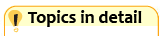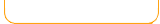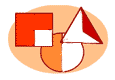Round and Estimate

 RoundEstimateRound Hundreds Estimate Numbers On Number LineRound numbers to the nearest hundred. Estimate numbers by locating them on a number line. Round Thousands Estimate Sums By Rounding Round numbers to the nearest thousand.Estimate sums by rounding each number to the nearest hundred then adding. Round Millions Estimate Differences By Rounding Round numbers to the nearest million. Estimate differences by rounding each number to the nearest hundred then subtracting.Round money amounts to the nearest dollar. Estimate products by rounding the first factor to the nearest ten then multiplying.Round decimals with Scooter Quest.Estimate fractions from the amount of shape colored. Round Mixed Numbers Estimate Integers On a Number LineRound mixed numbers to nearest whole number.Estimate products by rounding the first factor to the nearest ten then multiplying.